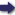(London :  Kegan Paul, Trench, Trübner & Co.,  1910.)

 Tools

## Search this bookPrev Page 312 Next``` 312 ALBERUNPS INDIA. On the cir¬ cumference of the earth. Page i6i. Quotations from the K/ianda- khddyaka and the Karana¬ tilaka. yojanas ; the more to the east it is, the smaller is this number. They call it desdntarct, i.e. the difference betioeen the places. Further, they multiply the desdntarct by the mean daily motion of the planet (the sun), and divide the product by 4800. Then the quotient repre¬ sents that amount of the motion of the star which corresponds to the number of yojctna in question, i.e. that which must be added to the mean place of the sun, as it has been found for moon or midnight of Ujain, if you want to find the longitude of the place in question. The number which they use as divisor (4800) is the number of the yojanas of the circumference of the earth, for the difference between the spheres of the meridians of the two places stands in the same relation to the whole circumference of the earth as the mean motion of the planet (sun) from one place to the other to its whole daily rotation round the earth. If the circumference of the earth is 4800 yojanas, the diameter is nearly 1527; but Pulisa reckons it as 1600, Brahmagupta as 1581 yojanas, each of which is equal to eight miles. The same value is given in the astro¬ nomical handbook Al-arkand as 1050. This number, however, is, according to Ibn Tarik, the radius, whilst the diameter is 2100 yojanas, each yojctna being reck¬ oned as equal to four miles, and the circumference is stated as 6596^^5- yojanas. Brahmagupta uses 4800 as the number of yojanas of the earth's circumference in his canon Khanda- khddyaka, but in the amended edition he uses, instead of this, the corrected circumference, agreeing with Pulisa. The correction he propounds is this, that he multiplies the yojanas of the earth's circumference by the sines of the complement of the latitude of the place, and divides the product by the sinus totus; then the quotient is the corrected circumference of the earth, or the number of yojanas of the parallel circle of the place in question. Sometimes this number is called the collar of the meri- ```Prev Page 312 Next